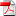# A Probabilistic Numerical Method for Optimal Multiple Switching Problem and Application to Investments in Electricity Generation

1
Oct

RR-FiME-12-07

### René Aïd, Luciano Campi, Nicolas Langrené, Huyên Pham

In this paper, we present a probabilistic numerical algorithm combining dynamic programming, Monte Carlo simulations and local basis regressions to solve non-stationary optimal multiple switching problems in infinite horizon. We provide the rate of convergence of the method in terms of the time step used to discretize the problem, of the size of the local hypercubes involved in the regressions, and of the truncating time horizon. To make the method viable for problems in high dimension and long time horizon, we extend a memory reduction method to the general Euler scheme, so that, when performing the numerical resolution, the storage of the Monte Carlo simulation paths is not needed. Then, we apply this algorithm to a model of optimal investment in power plants. This model takes into account electricity demand, cointegrated fuel prices, carbon price and random outages of power plants. It computes the optimal level of investment in each generation technology, considered as a whole, w.r.t. the electricity spot price. This electricity price is itself built according to a new extended structural model. In particular, it is a function of several factors, among which the installed capacities. The evolution of the optimal generation mix is illustrated on a realistic numerical problem in dimension eight, i.e. with two different technologies and six random factors.

•rr-fime-12-07